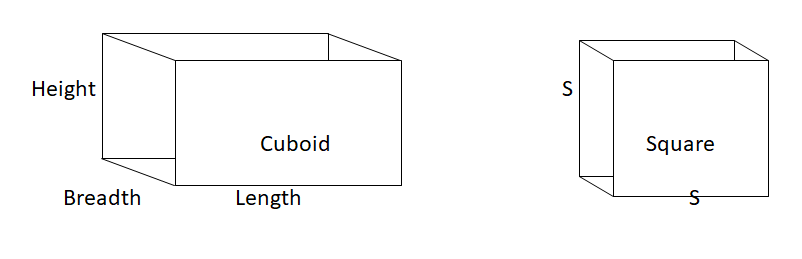Courses
Courses for Kids
Free study material
Offline Centres
MoreLast updated date: 09th Dec 2023
Total views: 384.3k
Views today: 8.84k

# A cubical box has each edge 10 cm and another cuboidal box is 12.5 cm long and 10 cm wide and 8 cm high. (i) Which box has the greater lateral surface area and by how much?(ii) Which box has the smaller total surface area and by how much?Verified
384.3k+ views
Hint- If a cube has an edge as $S$ then the lateral surface area of cube is given as $4{S^2}$ and the total surface area is given as $6{S^2}$ and if a cuboidal box has a length as $l$ , height as $h$ and width as $b$ then lateral surface area is given as $2h(l + b)$ and total surface area is given as $2(lb + bh + hl)$Given that cube has each edge $S = 10{\text{ cm}}$
And cuboidal box has length $l = 12.5{\text{ cm}}$ ,width $b = 10{\text{ }}{\text{cm}}$ , height $h = 8{\text{ cm}}$
Lateral surface area of cube $= 4{S^2}$
By putting the value of $S$ , we get
Lateral surface area of cube
$= 4 \times {10^2} \\ = 400{\text{ c}}{{\text{m}}^2} \\$
Lateral surface area of cuboidal box $= 2h(l + b)$
By putting the values of l, b, h, we get
Lateral surface area of cuboidal box
$= 2 \times 8 \times (12.5 + 10) \\ = 360{\text{ c}}{{\text{m}}^2} \\$
So, lateral surface area of cube is greater than cuboidal box
And the difference between their area is
$= 400 - 360 \\ = 40{\text{ c}}{{\text{m}}^2} \\$
Hence area difference is $= 40{\text{ c}}{{\text{m}}^2}$
Total surface area of cube is given as $6{S^2}$
Putting the value of edge in above equation, we get
Total surface area of cube
$= 6{S^2} \\ = 6 \times {10^2} \\ = 600{\text{ c}}{{\text{m}}^2} \\$
Total surface area of cuboidal box is given as $2(lb + bh + hl)$
Putting the value of length $l = 12.5{\text{ cm}}$ ,width $b = 10{\text{ }}{\text{cm}}$ , height $h = 8{\text{ cm}}$ above equation, we obtain
Total surface area of cuboidal box
$= 2(lb + bh + hl) \\ = 2(12.5 \times 10 + 10 \times 8 + 12.5 \times 8) \\ = 2(125 + 80 + 100) \\ = 610{\text{ c}}{{\text{m}}^2} \\$
Hence, the total surface area of cube is greater than the total surface area of cuboidal box
Therefore the area difference between them is $610 - 600 = 10{\text{ c}}{{\text{m}}^2}$

Note- These types of questions are based on formulas and to solve these types of questions you must remember the formulas. Try to remember the formulas with the help of diagrams and during solving the problem draw a rough diagram for your help. It helps in understanding the problem.